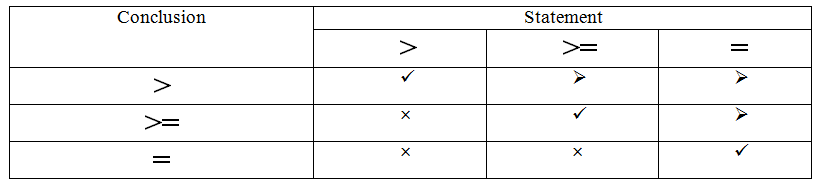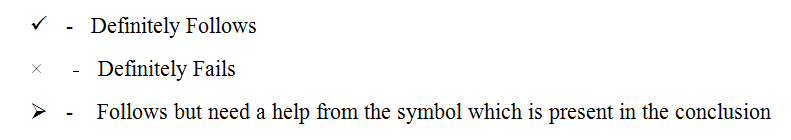# Can you solve Inequalities in less than 20 Sec :  Direct Inequalities Part 1

### Learn Series

Inequalities Introduction:-

This article covers the topic of Non Coded inequalities questions based on which are asked under the reasoning section in various bank examination. These types of questions are important for competitive exams like IBPS Clerk, SBI Clerk, SSC CGL, Placement Aptitude, IBPS PO, SBI PO, NICL AO, LIC AAO, SBI Associate Clerk, SBI Associate PO, CAT and others.

In Inequalities problems, you will be provided with a statement consisting of a group of elements. These elements will be having a certain relationship among them which is denoted by different inequality symbols like >, <, = etc.

You should know the meaning of different symbols which will help you in finding the relationships between the different elements of a statement.

In this article I’m going to explain you how to solve this inequalities problem within a minute by using open gate and close gate method.

Inequalities Points to remember:-

• Open Gate    >      Close Gate        –            Move from left to right
• Open Gate   >=    Open Gate        –            Move from left to right
• Open Gate    =      Open Gate        –            Move both direction
• Close Gate   <       Open Gate        –            Move from right to left
• Close Gate =<     Open Gate        –            Move from right to left

Step 1:  Check which entity has a open gate in conclusion.

Step 2:  Then starts move from the entity which has a open gate to another entity.

Step 3:  If we have close gates between the entities while moving from source to destination in statement, then that conclusion fails.

## Case (i) : Closed Gate

If you have a closed gate in your statement to reach your destination entity then your conclusions will fail. You don’t need to check further.

Eg:- Statement     : A>B>C<D

Conclusion    :  i) A>D   ii) C>A

Here, From the 1st conclusion we can clearly see that “A” has a open gate. Here ‘A’ & ‘D’ are ‘source’ and ‘destination’ entity respectively. Next check the statement is also having the open gate to reach D from A. But, In this case we have closed gate while trying to move from C to D. So, We can say that the 1st conclusion fails by checking the gates alone.

From the 2nd conclusion we can clearly see that “C” has a open gate. Here ‘C’ & ‘A’ are ‘source’ and ‘destination’ entity respectively. Next check the statement is also having the open gate to reach A from C. But, In this case we have closed gate while trying to move from C to B. So, We can say that the 2nd conclusion fails by checking the gates alone.

## Case(ii) : Open Gate

If you have open gate in the statement to reach your destination entity from source entity then you have to check the following rules:

Rule Box:From the above tabular coloumn you can easily understand the rules for all the relational symbols. We will discuss all the applicable rules in details are given below:

Inequalities Rule Explanation:

“> – Greater than”

1. If you have this symbol in your conclusion, Then it will satisfies all the symbols but “>” this symbol must present in your statement at least in one instance.
2. In this case also “=”,“>=” symbol follows the condition but “>” this symbol must present in your statement at least in one instance between your entities given in the conclusion.

Eg:-   Statement:  A>=B>=C;F=E=B>D

Conclusion:

i) A>C       False

ii) A>E      False

iii) B>F      False

iv) A>D      True

“Greater than or Equals  >=”

1. If you have this symbol in your conclusion, then it only satisfies these two symbols “>=”,”=” which is shown in the above tabular column.
2. In this case, “=” symbol follows the condition but “>=” this symbol must present in your statement at least in one instance between your entities given in the conclusion.

Eg:-  Statement: A>=B>=C;F<B=E=G

Conclusion:

i)   A>=F    False

ii) A>=E     True

iii)  B>=G   False

iv) A>=C     True

“Equal to (=)”

1. If you have this symbol in your conclusion, Then it only satisfies the “=” symbol which is  present in your statement between the entities in the given conclusion.
2. If rest of the symbols are present in between your entities then your conclusion will fails.

Eg:-   Statement:  A=B>=C;E=B>F

Conclusion:

i) A=C         False

ii) A=F        False

iii) A=E        True

Inequalities Solved Problems:-

Example 1:  Statement   :  A>=B>C>D;E<B

Conclusion :  i) A>E

1. Here, we have open gate for the entity “A”. So you can start moving from A to E.
2. Further, we have to check whether the given statement has open gate from source to destination i.e.from A to E. And also check the rules table for the “>” symbol.
3. Here we have open gate from source to destination and rule table also follows the statement. Hence, this conclusion follows the statement.

Example 2:   Statement :   A>=C>B>D;P<F>=C>E

Conclusion:  i)  F>D

1. Here, we have open gate for the entity “F”. So you can start moving from F to D.
2. Next, we have to move from source ‘F’ to destination entity ‘D’.
• First, move F to C after that we can jump to the C which is present in the first part from that C move to B and D as well.
1. Once you reached your destination, Rule box states that if you have this “>” symbol in your conclusion then we must have this “> or <” symbol in between the source and destination on the statement.
2. Yes we are having this “> or <” Symbol in the statement. Hence, this conclusion follows the statement.

Other Posts you may Like